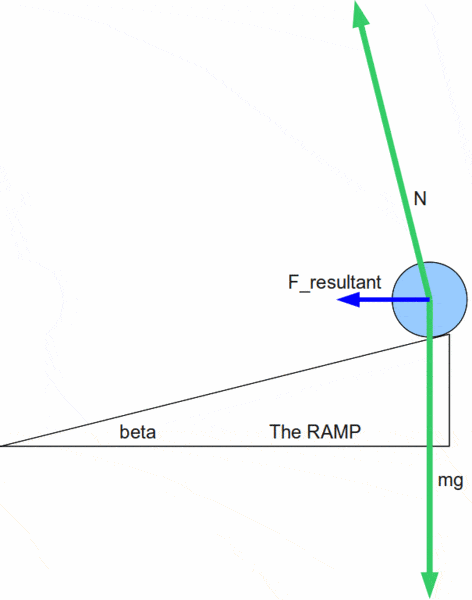# Angle for a banked curve (friction-less surface)

## Homework Statement

At what angle should the roadway on a curve with a 50 m radius be banked to allow cars to negotiate the curve at 12 m/s even if the roadway is frictionless?

a. 0°
b. 12.2°
c. 17.1°
d. 35.0°
e. 73.2°

## Homework Equations

β=arctan(v^2/r*g)

## The Attempt at a Solution

im using β=arctan(v^2/r*g) but the key says the answer is b?
can anyone help me figure out why its not 16.4??

Why not try to derive the formula?

i did...i though of the triangle as if the gravity was pointing downward the centripetal force outward and then the angle should be the same as the angle for the curve right?

If your acceleration is towards the centre the force will be too. You need to find the angle of the road that gives a normal force with the horizontal component equal to the force needed to keep the circular motion described by v = 12 m/s and r = 50m

I'm aware that my response is a mouthful, feel free to ask me for clarification.

do you mean like a triangle with the vertical 9.81*m and the horizontal (12^2/50)*m ??

do you mean like a triangle with the vertical 9.81*m and the horizontal (12^2/50)*m ??

Yes. But the horizontal component will be a fraction of the normal force, which depends on the angle.

I like Serena
Homework Helper
Welcome to PF, physgrl!I derived the same formula as you have.
When I fill in the values I also get 16.4 degrees.
So I believe your answer is correct (assuming you did not make a typo).

Welcome to PF, physgrl!I derived the same formula as you have.
When I fill in the values I also get 16.4 degrees.
So I believe your answer is correct (assuming you did not make a typo).

I believe your answer is incorrect, as I got 17.1 as my answer. You should investigate why you are using arctan.

I like Serena
Homework Helper
I believe your answer is incorrect, as I got 17.1 as my answer. You should investigate why you are using arctan.

Here's my interpretation of the forces.We have:
$$F_{resultant} = {m v^2 \over r}$$
$$\tan \beta = {F_{resultant} \over m g }$$
The OP's formula follows from this.

#### Attachments

ehild
Homework Helper
16.4° is correct, the answer key is wrong. It happens quite often.

ehild

Oooh I see where I went wrong. My apologies to all. I just always have trouble with the normal force being more than gravity. Its very unintuitive to me.

Thanks to everyone!!

I like Serena
Homework Helper
You're welcome!